Courses

RD Sharma Solutions (Part - 2) - Ex-13.1, Simple Interest, Class 7, Math Notes | Study RD Sharma Solutions for Class 7 Mathematics - Class 7

Class 7: RD Sharma Solutions (Part - 2) - Ex-13.1, Simple Interest, Class 7, Math Notes | Study RD Sharma Solutions for Class 7 Mathematics - Class 7

The document RD Sharma Solutions (Part - 2) - Ex-13.1, Simple Interest, Class 7, Math Notes | Study RD Sharma Solutions for Class 7 Mathematics - Class 7 is a part of the Class 7 Course RD Sharma Solutions for Class 7 Mathematics.
All you need of Class 7 at this link: Class 7

QUESTION 12:

Mr Garg lent Rs 15000 to his friend. He charged 15% per annum on Rs 12500 and 18% on the rest. How much interest does he earn in 3 years?

Principal amount (P) = Rs 12500
Time period (T) = 3 years
Rate of interest (R)  = 15% p.a.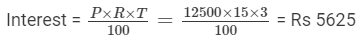Rest of the amount lent =  Rs 15000 − Rs 12500 = Rs 2500
Rate of interest = 18 % p.a.
Time period = 3 years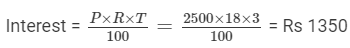Total interest earned = Rs 5625 + Rs 1350 = Rs 6975

Question 13:

Shikha deposited Rs 2000 in a bank which pays 6% simple interest. She withdrew Rs 700 at the end of first year. What will be her balance after 3 years?

Principal amount deposited  (P) = Rs 2000
Time period (T) = 1 year
Rate of interest (R)  = 6% p.a.

Interest after 1 year =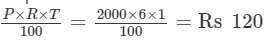So amount after 1 year = Principal amount + Interest = 2000 + 120 = Rs 2120
After 1 year, amount withdrawn = Rs 700
Principal amount left (P1) = Rs 2120 − Rs 700 = Rs 1420
Time period (T) = 2 years
Rate of interest (R)  = 6% p.a.

Interest after 2 years =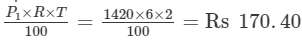Total amount after 3 years = Rs 1420  + Rs 170.40 = Rs 1590.40

Question 14:

Reema took a loan of Rs 8000 from a money lender, who charged interest at the rate of 18% per annum. After 2 years, Reema paid him Rs 10400 and wrist watch to clear the debt. What is the price of the watch?

Principal amount (P) = Rs 8,000
Rate of interest (R) = 18%
Time period (T) = 2 years

Interest after 2 years =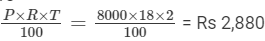Total amount payable by Reema after 2 years = Rs 8,000 + Rs 2,880 = Rs 10,880
Amount paid = Rs 10,400
Value of the watch = Rs 10,880 − Rs 10,400 = Rs 480

Question 15:

Mr Sharma deposited Rs 20000 as a fixed deposit in a bank at 10% per annual. If 30% is deducted as income tax on the interest earned, find his annual income.

Amount deposit (P) = Rs 20,000
Rate of interest (R) = 10% p.a.
Time period (T) = 1 year

Interest after 1 year =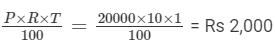Amount deducted as income tax = 30% of Rs 2,000 =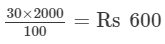Annual interest after tax deduction = Rs 2,000 − Rs 600 = Rs 1,400

The document RD Sharma Solutions (Part - 2) - Ex-13.1, Simple Interest, Class 7, Math Notes | Study RD Sharma Solutions for Class 7 Mathematics - Class 7 is a part of the Class 7 Course RD Sharma Solutions for Class 7 Mathematics.
All you need of Class 7 at this link: Class 7Use Code STAYHOME200 and get INR 200 additional OFF Use Coupon Code

Top Courses for Class 7RD Sharma Solutions for Class 7 Mathematics

97 docs

Top Courses for Class 7Track your progress, build streaks, highlight & save important lessons and more!

,

,

,

,

,

,

,

,

,

,

,

,

,

,

,

,

,

,

,

,

,

,

,

,

,

,

,

,

,

,

;